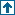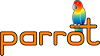parrotcode: Draw a circle on any VT100-compatible screen Contents | IMCC

# NAMEexamples/pir/circle.pir - Draw a circle on any VT100-compatible screen

# SYNOPSIS`    % ./parrot examples/pir/circle.pir`

# DESCRIPTIONThis draws an ASCII-art representation of a circle (or oval) using only integer arithmetic and no trig.

The algorithm is based on:

```  cos(theta+delta) =
cos(theta) -
[alpha*cos(theta) + beta*sin(theta)]```

and

```  sin(theta+delta) =
sin(theta) -
[alpha*sin(theta) - beta*cos(theta)]```

where

`  alpha = 2*sin^2(delta/2) and beta = sin(delta)`

(See Numerical Recipes in C / The Art of Scientific Computing)

In this program the value of delta is chosen to be 1/(1<<n) such that it is small enough that

• alpha is approximately 0
• beta is approximately delta

# AUTHORWritten for parrot by Nick Glencross <nick.glencross@gmail.com>. Adapted from a machine code program that I wrote for a BBC micro.

# COPYRIGHTSame as parrot.